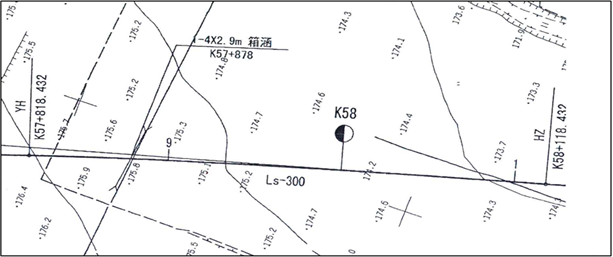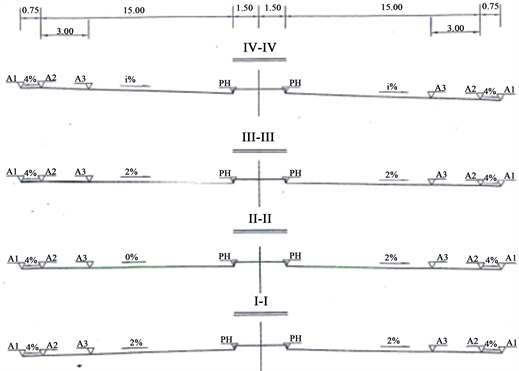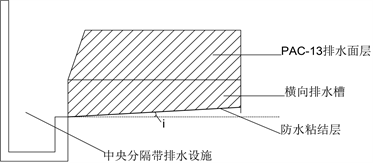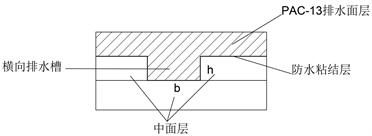#### 期刊菜单

Design Method for Air Void and Internal Drainage of Porous Asphalt Pavement at Highway Superelevation Transition Section
DOI: 10.12677/HJCE.2022.119111, PDF , HTML, XML, 下载: 156  浏览: 272

Abstract: The superelevation transition section of the highway is highly susceptible to water on the roadway due to the low longitudinal and transverse slopes. In order to solve the problem of water on the road surface of the superelevation transition section, the design of porous asphalt pavement was proposed based on a pavement project of a superelevation transition section of Qinglin Expressway. Firstly, the calculation method of air void under two working conditions of no road surface water and water film thickness less than critical water film thickness is given with engineering cases. Then, a transverse drainage channel structure matching the internal drainage of the porous asphalt pavement is proposed, and a method for determining the size and number of transverse drainage channels is given. The results of the case calculations show that the target air void of porous asphalt pavement and the design method of internal drainage facilities given in this paper are feasible and reasonable, which can provide a reference for solving the problem of water on the road surface of the superelevation transition section of highway.

1. 引言

2. 工程案例概况Figure 1. Route plan of the waterlogged sectionFigure 2. Superelevation transition section transition approach

$q=\frac{4843.466×\left(1+0.984\mathrm{lg}P\right)}{{\left(t+19.481\right)}^{0.932}}$ (1)

${q}_{p,t}=\frac{1000×60}{1×10000×1000}=6×{10}^{-3}q$ (2)

3. 目标空隙率确定

3.1. 路面无积水

${W}_{饱}=\frac{h{C}_{rw}\sqrt{{i}_{z}^{2}+{i}_{h}^{2}}}{100L\sqrt{1+\frac{{i}_{z}^{2}}{{i}_{h}^{2}}}}$ (3)

${C}_{rw}$ ——排水沥青混合料透水系数(cm/s)；

$h$ ——排水面层(排水功能层)厚度(cm)：

${i}_{z}$ ——纵坡坡度(%)；

${i}_{h}$ ——横坡坡度(%)；

$L$ ——单向路面宽度(m)，对于高速公路为半幅路面宽度。

${C}_{rw}=\frac{100{W}_{饱}L\sqrt{1+\frac{{i}_{z}^{2}}{{i}_{h}^{2}}}}{h\sqrt{{i}_{z}^{2}+{i}_{h}^{2}}}$ (4)

${C}_{rw}=0.0372{\text{e}}^{16.379n}$ (5)

3.2. 路面水膜厚度小于临界水膜厚度

${h}_{轮}=1.3589{\left[\frac{\left(100WL-kh\sqrt{{i}_{z}^{2}+{i}_{h}^{2}}\right)nl}{{\left({i}_{z}^{2}+{i}_{h}^{2}\right)}^{\frac{1}{4}}}\right]}^{\frac{3}{5}}$ (6)

${h}_{临}=1000\left[\frac{G}{\left(\sqrt{2}-1\right)\rho w{v}^{2}{r}^{\frac{1}{2}}}\right]$ (7)

${h}_{临}$ ——临界水膜厚度(mm)；

$W$ ——降雨强度(cm/s)；

$L$ ——单向路面宽度(m)，对于高速公路为半幅路面宽度；

$k$ ——渗透系数，常数；

$l$ ——最外侧车行道右侧轮迹带距离路面左边缘距离(m)；

$n$ ——粗糙系数，经验常数，排水沥青路面一般取0.03；

$G$ ——车重(N)，本设计取500 kN；

$\rho$ ——水的密度(kg/m3)，取值1000；

$w$ ——车胎宽度(m)，本设计取0.2；

$v$ ——车速(m/s)，本设计取33.3；

$r$ ——轮胎半径(m)，本设计取0.3。

$n=\sqrt[5.6]{\frac{k{S}_{Agg}^{5.1}\mu }{2\gamma }}$ (8)

$\gamma$ ——水的重度，当水温为20℃时， $\gamma =9.79\text{}\text{kN}/{\text{m}}^{3}$

${S}_{Agg}$ ——集料的比表面积(mm−1)。

4. 排水路面内部排水设施设计(a)(b)

Figure 3. Schematic diagram of the lateral drainage facilities. (a) Longitudinal section; (b) Cross-sectional view

4.1. 设计径流量

$Q=16.67\psi {q}_{p,t}F$ (9)

${t}_{2}=\frac{l}{60v}$$l$ 为切槽长度； $v=20{i}^{0.6}$。所以，降雨历时 $t=5+{t}_{2}$

$Q=15.8365{q}_{p,t}LB=95.019×\frac{4.843466×\left(1+0.984\mathrm{lg}5\right)}{{\left(24.481+\frac{l}{1200{i}^{0.6}}\right)}^{0.932}}LB=\frac{776.75526}{{\left(24.481+\frac{l}{1200{i}^{0.6}}\right)}^{0.932}}LB$ (10)

4.2. 横向排水槽排水能力

$Q=AC\sqrt{Ri}$ (11)

$Q=AC\sqrt{Ri}=\frac{{\left(hb\right)}^{\frac{5}{3}}\cdot \sqrt{i}}{n\cdot {\left(b+2h\right)}^{\frac{2}{3}}}$ (12)

4.3. 工程案例

5. 结论

1) 通过工程案例给出了排水沥青路面目标空隙率的确定方法，即按照路面无积水与路面水膜厚度小于临界水膜厚度两种工况计算，最终采用的空隙率取决于公路所处的实际工况与业主要求。

2) 提出了匹配超高缓和段排水沥青路面内部排水设施，即在中面层顶部开设横向排水槽。横向排水槽的尺寸可根据设计径流量和排水能力的计算模型合理设计。

3) 结合青临高速工程案例，具体阐述了PAC空隙率和横向排水槽设计方法，对解决超高缓和段路面积水难题具有一定的借鉴意义。

  张爱花, 牛肖. 多雨区高速公路超高过渡段路面积水处理措施研究[J]. 公路交通科技(应用技术版), 2020, 16(8): 32-33, 39.  祁颖智. 基于道路线形组合的路面径流行为研究[D]: [硕士学位论文]. 南京: 东南大学, 2019.  杨梦, 汤天乐, 胡琦. 排水沥青路面处治超高渐变段路面积水问题的应用研究[J]. 湖南交通科技, 2021, 47(3): 58-62.  张锋, 刘永旭, 杜晓博. 高速公路超高渐变段排水技术研究[J]. 华东公路, 2019(1): 44-48.  余军, 余晓敏. 超高过渡段上的积水和其振动标线的设置[J]. 江西建材, 2013(5): 230-231.  Chen, J.-S. and Yang, C.H. (2021) Porous Asphalt Concrete: A Review of Design, Construction, Performance and Maintenance. International Journal of Pavement Research and Technology, 13, 601-612. https://doi.org/10.1007/s42947-020-0039-7  张燕飞. 降雨条件下多车道高速公路超高过渡段交通安全保障技术研究[D]: [硕士学位论文]. 西安: 长安大学, 2020.  于良溟. 排水性沥青路面防水粘结层研究[D]: [硕士学位论文]. 南京: 东南大学, 2006.  沈安琪. PAC-13排水路面在南方多雨地区高速公路“零坡段”的应用[J]. 广东公路交通, 2021, 47(5): 5-10.  Kuruppu, U., Rahman, A. and Rahman, M.A. (2019) Permeable Pavement as a Storm Water Best Management Practice: A Review and Discussion. Environmental Earth Sciences, 78, Article No. 327. https://doi.org/10.1007/s12665-019-8312-2  肖鑫. 排水沥青混合料细观结构及排水特性研究[D]: [博士学位论文]. 广州: 华南理工大学, 2014.  肖鑫, 张肖宁. 排水沥青路面排水能力分析及目标空隙率确定[J]. 中外公路, 2016, 36(1): 49-53.  马翔, 倪富健, 李强. 排水面层渗流模型及参数[J]. 东南大学学报(自然科学版), 2014, 44(2): 381-385.  汪鸿山, 冯德成, 解晓光. 孔隙率对透水性沥青路面渗透性能的影响规律[J]. 建筑材料学报, 2017, 20(3): 464-468.  潍坊市气象局. 关于发布潍坊市中心城区暴雨强度公式(修订)的通告[EB/OL]. http://csglj.weifang.gov.cn/SZDT/TZGG/201511/t20151112_1613002.htm, 2015-11-12.  中交路桥技术有限公司. JTG/T D33-2012, 公路排水设计规范[S]. 北京: 人民交通出版社, 2013.  交通运输部公路交通研究院. JTG/T 3350-03-2020, 排水沥青路面设计与施工技术规范[S]. 北京: 人民交通出版社, 2020.  纪洋洋. 排水路面渗流模型分析与试验研究[D]: [硕士学位论文]. 南京: 南京航空航天大学, 2018.  Luo, W. and Li, L. (2019) Development of a New Analytical Water Film Depth (WFD) Prediction Model for Asphalt Pavement Drainage Evaluation. Construction and Building Materials, 218, 530-542. https://doi.org/10.1016/j.conbuildmat.2019.05.142